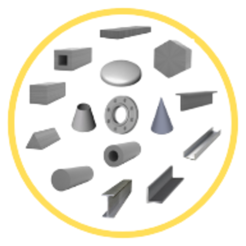## Screenshots

•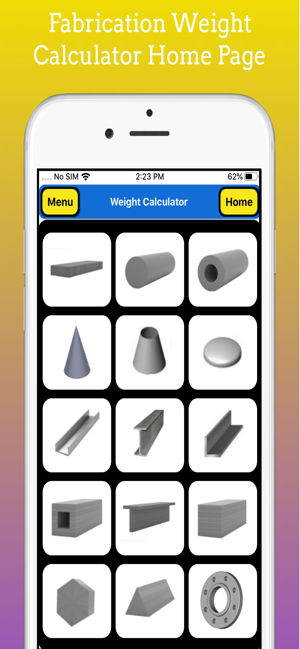•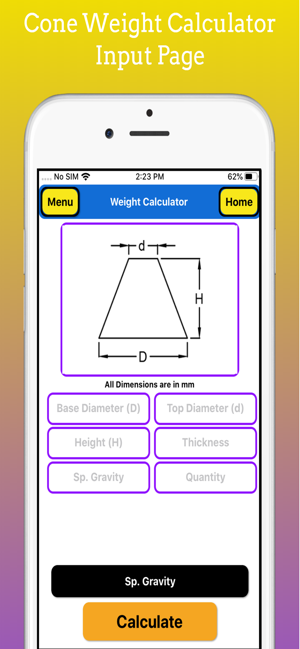•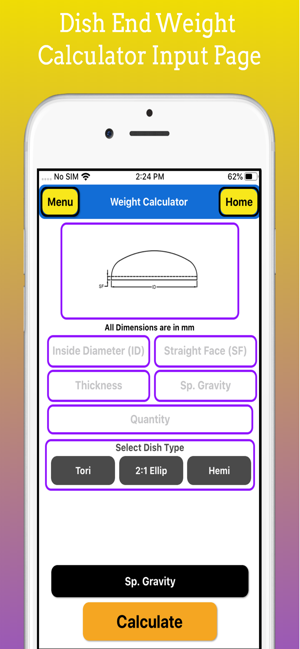•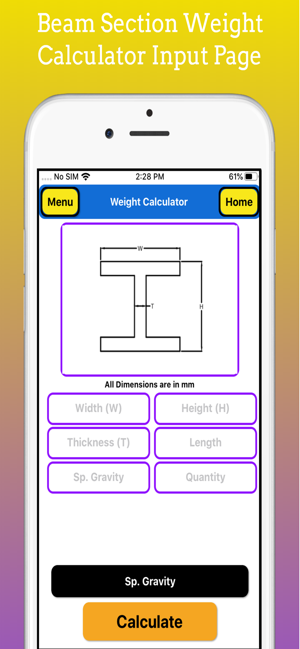•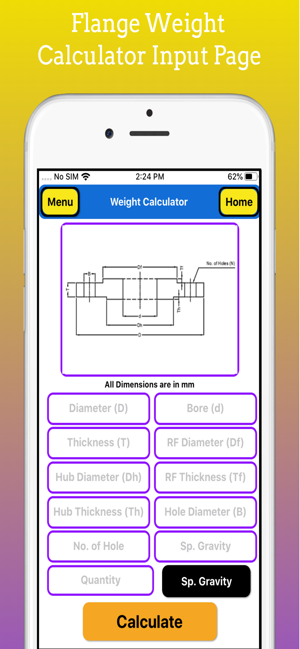•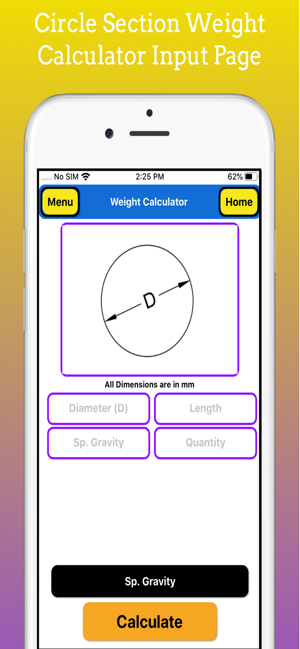•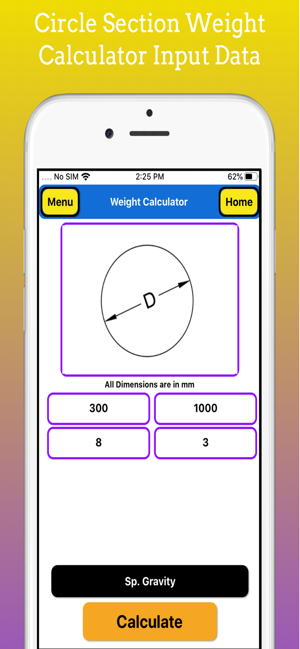•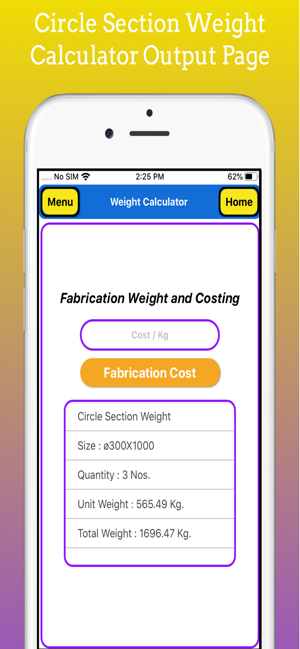•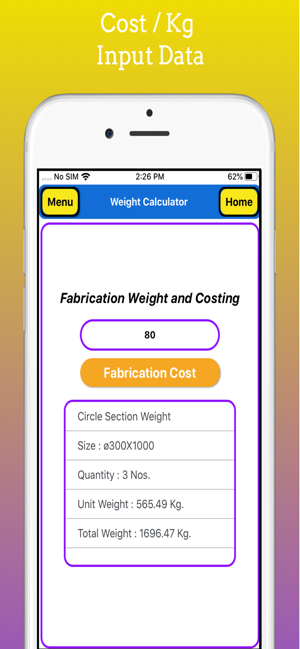•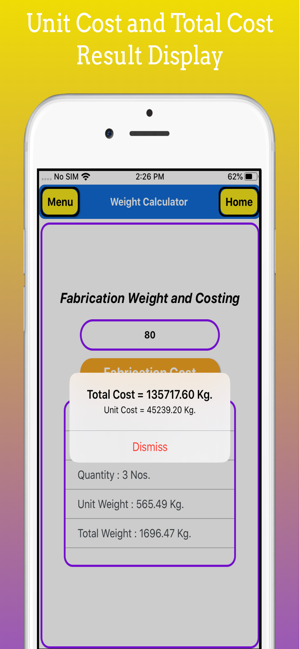## Description

This app is build to calculate fabrication weight and costing of various shapes which are widely used in fabrication of Process equipment, pressure vessels and Structural Fabrication.

Its has Fabrication Weight Calculation of Plate, Pipe, Circle, Cone, Dish, Angle, Channel, Beam, Tee, Square Bar, Hex Bar, Triangular Bar, Flange.

Metal Weight Calculator is a fast and simple app for calculating weights of metals.

In this App Following Weight Calculator is Available :

1. Plate Section Weight Calculator
2. Circle Section Weight Calculator
3. Pipe or Shell Section Weight Calculator
4. Full Cone Section Weight Calculator.
5. Truncated Cone or Half Cone Weight Calculator
6. Dish Ends Section Weight Calculator.
7. Channel Section Weight Calculator
8. Beam Section Weight Calculator
9. Angle Section Weight Calculator
10. Square Tube Section Weight Calculator
11. Tee Section Weight Calculator
12. Square Bar Section Weight Calculator
13.Hex Bar Section Weight Calculator
14. Triangular Bar Section Weight Calculator
15. Flange Section Weight Calculator.

It Supports All Material some of common material as Carbon Steel, Stainless Steel, Aluminium, Copper, Brass, Bronze, Cast Iron, Lead etc.

You Can Calculate Weight of Any Engineering Material by giving input as Specific Gravity of this Material.

This app is also called as metal weight calculator, engineering eight calculator., Steel Weight Calculator, Metal Weight Calculator.

This app calculate Weight of All Shapes used in fabrication such as Plate, Shell, Circle or Round bar, Pipe, Dish Ends, Full Cone, Truncated Cone, Angle, Channel, Beam, Hex Bar ,Triangular bar, Square bar, Square Tube, Rectangular Tube , Flange etc.

“Fabrication Weight Calculator” is a simple application developed to simplify the task of theoretical calculation of mass and cost of standard engineering/structural components

In this app all dimension are required in mm or metric system.

This app is useful for professional work in the field of fabrication of Process equipment's, such as pressure vessel, Heat Ex changers, Storage tank, Piping, Structures or which are in engineering services.

## Information

Provider
Imran Pinjara
Size
64.5 MB
Category
Utilities
Compatibility

Requires iOS 10.0 or later. Compatible with iPhone, iPad and iPod touch.

Languages

English

Age Rating
4+
Price
Free

## Supports

•